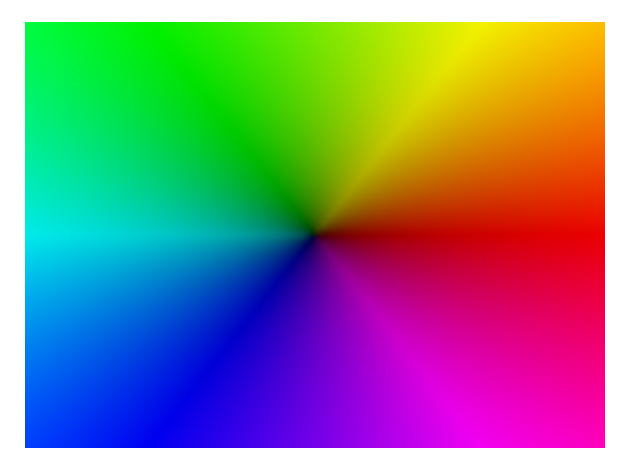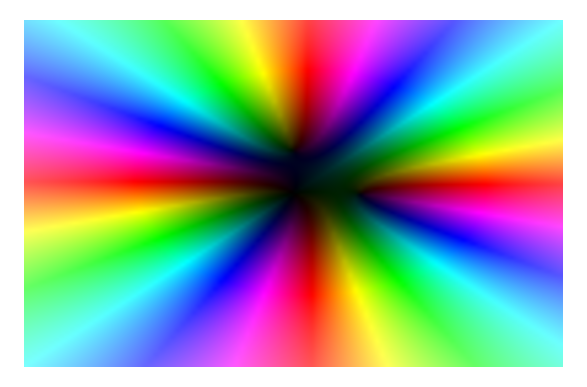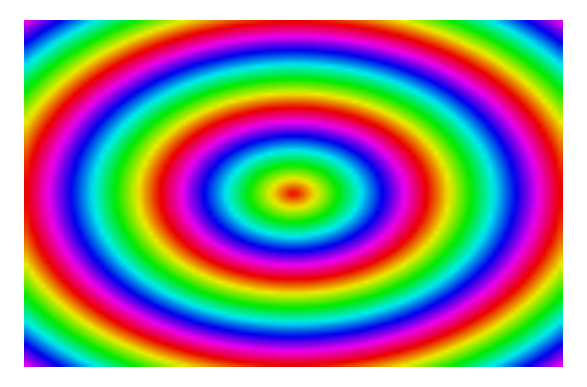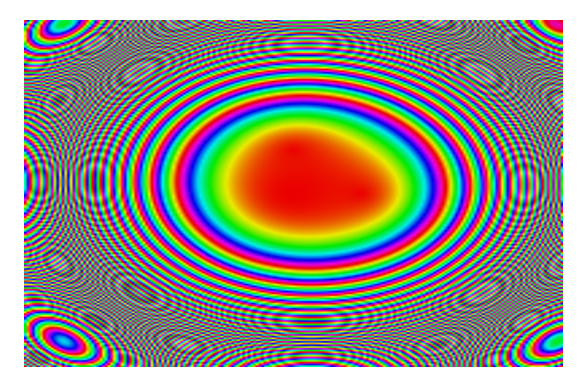# mixedmath

Explorations in math and programming
David Lowry-Duda

# Making plots of modular forms¶

Inspired by the images and ideas of Elias Wegert, I thought it might be interesting to attempt to implement a version of his colorizing technique for complex functions in sage. The purpose is ultimately to revisit how one plots modular forms in the LMFDB (see lmfdb.org and click around to see various plots — some are good, others are less good).   The challenge is that plotting a function from $\mathbb{C} \longrightarrow \mathbb{C}$ is that the graph is naturally 4-dimensional, and we are very bad at visualizing 4d things. In fact, we want to use only 2d to visualize it. A complex number $z = re^{i \theta}$ is determined by the magnitude ($r$) and the argument ($\theta$). Thus one typical approach to represent the value taken by a function $f$ at a point $z$ is to represent the magnitude of $f(z)$ in terms of the brightness, and to represent the argument in terms of color. For example, the typical complex space would then look like the following.
In :
P = complex_plot(x, [-2, 2], [-2, 2])
P.axes(False)
P.show()To get an idea for a slightly more complicated plot, we might look at $z^2(z-0.5)(z-0.5i)$
In :
complicated_func = x**2 * (x - 0.5) * (x - 0.5*i)

In :
P = complex_plot(complicated_func, [-2, 2], [-2, 2])
P.axes(False)
P.show()Sometimes one chooses to plot only the magnitude or the argument. By discarding the argument, one can use cycling colors to represent changing magnitudes. For example, one could have red represent 0, and as magnitude increases the colors might rotate through purple, blue, green, yellow and orange, and eventually cycle to represent more magnitudes.
In :
def normalize(complexval):
return 2*exp(2 * pi * i * complexval.abs())

In :
f = lambda x: normalize(x)
P = complex_plot(f, [-2, 2], [-2, 2])
P.axes(False)
P.show()Doing this with our slightly more complicated function gives
In :
f = lambda x: normalize(complicated_func(x))
P = complex_plot(f, [-2, 2], [-2, 2], plot_points=200)
P.axes(False)
P.show()

/home/davidlowryduda/sagebuild/sage2/local/lib/python2.7/site-packages/sage/misc/decorators.py:493: DeprecationWarning: Substitution using function-call syntax and unnamed arguments is deprecated and will be removed from a future release of Sage; you can use named arguments instead, like EXPR(x=..., y=...)
See http://trac.sagemath.org/5930 for details.
return func(*args, **options)The fact that there are three zeros in the central red blob is lost. It's not particularly illuminating. We can change this a bit by changing how often the colors cycle. Instead of cycling every time the magnitude increases by a multiple of $2\pi$, we could have it change every time the magnitude increases by a factor of $e$ (or whatever other number).
In :
def normalize_log(complexval):
return 2*exp(2 * pi * i * log(complexval.abs()))

In :
f = lambda x: normalize_log(x)
P = complex_plot(f, [-2, 2], [-2, 2], plot_points=200)
P.axes(False)
P.show()